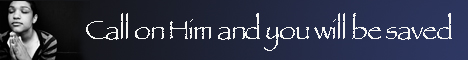Note:  Do not rely on this information. It is very old.

# Parallelogramof Forces

Parallelogram of Forces follows from Newton's second law of motion (q.v.). If two forces acting at any point B may be represented in magnitude and direction by the lines B A, B D, and if the parallelogram A B D C be completed, its diagonal B C will represent in magnitude and direction the resultant of those two forces. This proposition can also be deduced from the parallelogram of velocities (q.v.), since a force is equal to the product of a mass and an acceleration, and an acceleration is the velocity generated per second.